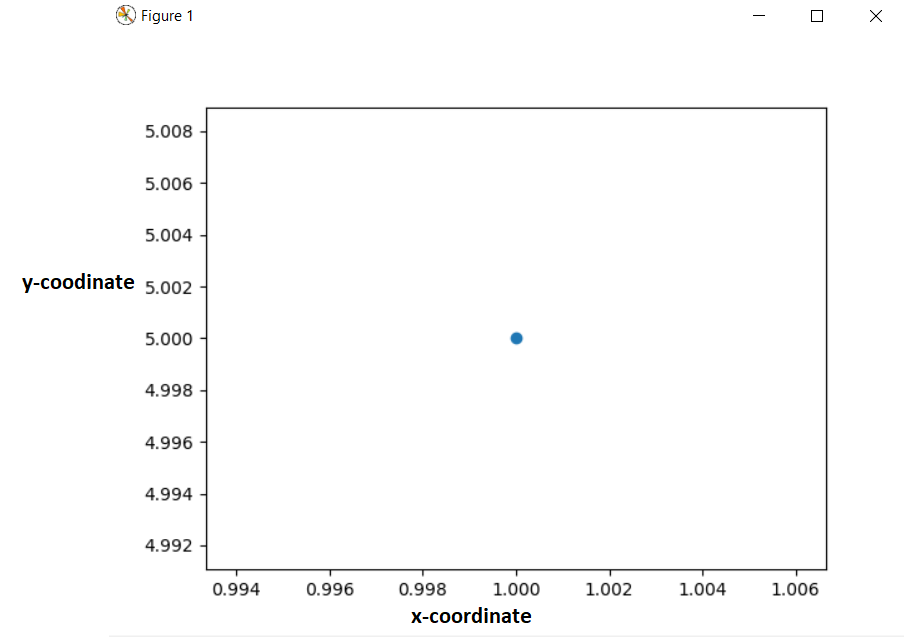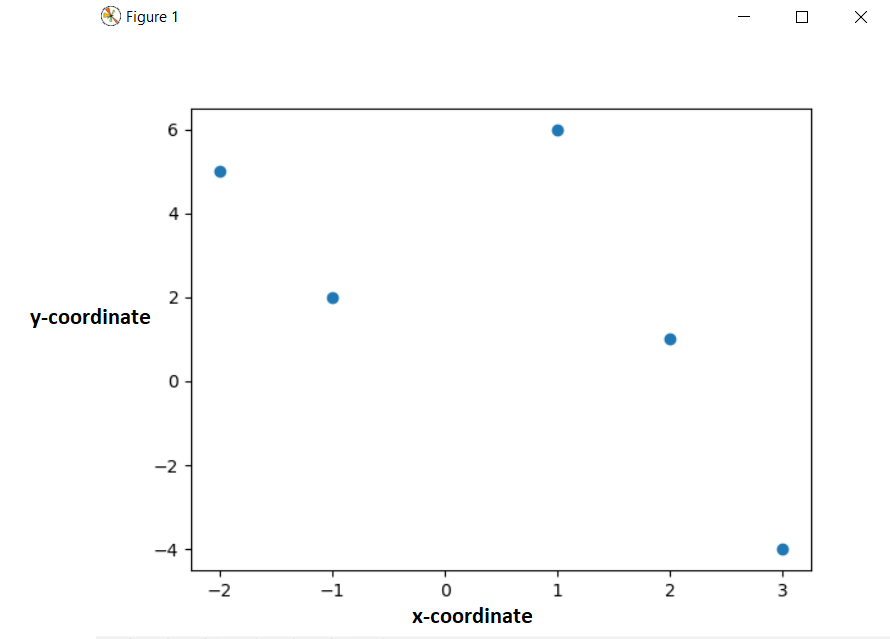# How to plot points in matplotlib with Python

In this article, we will learn “How to plot points in matplotlib with Python”. For this, we have to implement two popular modules of Python in the field of plotting graph or figure named “matplotlib” and “numpy“. The main motto of this article is to learn how to plot any point or any graph(scattered point of graph) in matplotlib using Python.

## Plotting of points in matplotlib with Python

There is a method named as “scatter(X,Y)” which is used to plot any points in matplotlib using Python, where X is data of x-axis and Y is data of y-axis.

Let’s understand this with some example:-

• In this example, we will plot only one point

```# importing two required module
import numpy as np
import matplotlib.pyplot as plt

# Creating a numpy array
X = np.array()
Y = np.array()

# Plotting point using sactter method
plt.scatter(X,Y)
plt.show()```

Output:-In the above example, the first step is to import two modules of Python named as numpy and matplotlib by these two lines of codes:-

1. import numpy as np
2. import matplotlib.pyplot as plt

and then we created a numpy array and stored in a variable named as X and then created another numpy array and stored this in another variable named as Y. We stored only one value in X and Y, since we have to plot a single point in this example. Then we used the “plt.scatter(X,Y)” and “plt.show()” to plot that required point.

• In this example, we will plot many points.
```# importing two required module
import numpy as np
import matplotlib.pyplot as plt

# Creating a numpy array
X = np.array([1,2,3,-1,-2])
Y = np.array([6,1,-4,2,5])

# Plotting point using scatter method
plt.scatter(X,Y)
plt.show()```

Output:-The explanation for the above example is the same as the first example, the only difference is that we stored more than one variable in X and Y, since we have to plot more than one point.

• In this example, we will plot a sine function point.
```# importing two required module
import numpy as np
import matplotlib.pyplot as plt

# Taking points on x-axis from 0 to 10 and the last argument 30 is stating that 10 is divided into thirty equal interval.
x = np.linspace(0,10,30)

# y is a sine function
y = np.sin(x)

# Plotting point using scatter method
plt.scatter(x, y,color="black")
plt.show()```

Output:-In the above example again the explanation is the same as explained above, the only difference is that we used a new method “np.linspace(0,10,30)“. This method is used to divide an equal interval between two points.

Let’s understand with an example:-

Let say we used “np.linspace(0,10,30)” this means that we are dividing 0-10 interval into 30 equal interval. So, there are 30 points located in above plot.

You may also read these related articles:-

### One response to “How to plot points in matplotlib with Python”

1. leila says:

ok imagine i plot one figure by point- point method like 4 point x and 4 point y but i woyld like on y axes show 10 point how can i give range to y axis because in point by point the number of x and y points should be equal?